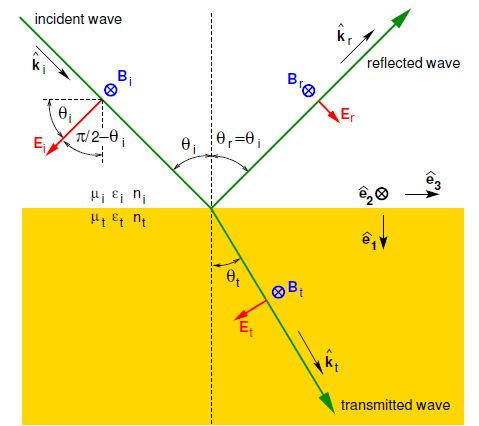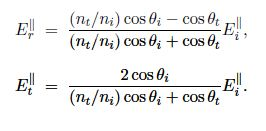# Amplitude Reflection and Transmission Coefficient for Parallel Polarization

Amplitude reflection and transmission coefficient for parallel polarizationFigure: EM field geometry for parallel (π, p) polarisation. The magnetic field is shown pointing in to the page.

The EM field geometry for parallel (π, p) polarisation is shown in Figure. In this case, B • e1 = 0, B • e3 = 0 and E • e2 = 0, and so the three simultaneous equations remaining areSolving simultaneous Equations we obtain;Using Snell’s law to replace θt we arrive at the amplitude reflection coefficient for parallel polarisation,and the amplitude transmission coefficient for parallel polarisation,